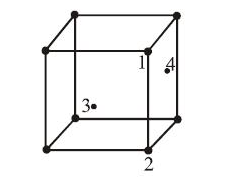CsCl crystallises in body-centred cubic lattice. If 'a' is its edge length then which of the following expressions is correct?

(a) rCs+ + rCl- = 3a

(b)  rCs+ + rCl- = 3a/2

(c)  rCs+ + rCl- = ($\sqrt{3}$/2)*a

(d) rCs+ + rCl-$\sqrt{3a}$

Concept Questions :-

Introduction and Type of Crystal System
High Yielding Test Series + Question Bank - NEET 2020

Difficulty Level:

In an f.c.c. unit cell. atoms are numbered as shown below.The atoms not touching each other are (Atom numbered 3 is face centre of front face).(A) 3&4
(B) 1&3
(C) 1&2
(D) 2&4

Concept Questions :-

Introduction and Type of Crystal System
High Yielding Test Series + Question Bank - NEET 2020

Difficulty Level:

Which is covalent solid?

(a) Fe2O3

(b) Diamond

(c) Graphite

(d) All of these

Concept Questions :-

Introduction and Type of Crystal System
High Yielding Test Series + Question Bank - NEET 2020

Difficulty Level:

The mass of a unit cell of CsCl corresponds to:-

(a)8Cs+ and Cl-

(b)1Cs+ and 6Cl-

(c)1Cs+ and 1Cl-

(d)4Cs+ and Cl-

Concept Questions :-

Introduction and Type of Crystal System
High Yielding Test Series + Question Bank - NEET 2020

Difficulty Level:

Graphite in an example of-

(A) Ionic solid

(B) Covalent solid

(C) Vander waal's crystal

(D) Metallic crystal

Concept Questions :-

Introduction and Type of Crystal System
High Yielding Test Series + Question Bank - NEET 2020

Difficulty Level:

The intermetallic compound LiAg has a cubic crystalline structure in which each Li atom has 8 nearest neighbour silver atoms and vice-versa. What is the type of unit cell?

1. Body centred cubic

2. Face centred cubic

3. Simple cubic for either Li atoms alone or Ag atoms alone

4. None of the above

Concept Questions :-

Introduction and Type of Crystal System
High Yielding Test Series + Question Bank - NEET 2020

Difficulty Level:

How many octahedral and tetrahedral holes are present per unit cell in a face centred cubic arrangement of atoms?

(A) 8,4

(B) 1.2

(C) 4.8

(D) 2.1

Concept Questions :-

Introduction and Type of Crystal System
High Yielding Test Series + Question Bank - NEET 2020

Difficulty Level:

TiO2 is well known example of:

(a) triclinic system

(b) tetragonal system

(c) monoclinic system

(d) none of these

Concept Questions :-

Introduction and Type of Crystal System
High Yielding Test Series + Question Bank - NEET 2020

Difficulty Level:

Which pairs shows isomorphism?
(a) KNO3, NaNO3
(b) Cr2O3, FeO
(c) Both (a) and (b)
(d) Ferrimagnetic

Concept Questions :-

Introduction and Type of Crystal System
High Yielding Test Series + Question Bank - NEET 2020

Difficulty Level:

The elements of symmetry in a crystal are:-
(a) plane of symmetry
(b) axis of symmetry
(c) centre of symmetry
(d) all of these

Concept Questions :-

Introduction and Type of Crystal System